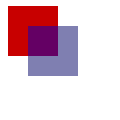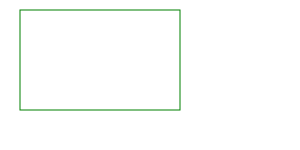If you read my post on “How to write a basic markdown editor with canvas”, you should now have a basic way to write some text and headings into a canvas-rendered editor. In this post, we’re going to continue our work with the canvas api to add support for embedding code blocks. We’ll make use of a few more canvas functions to render some custom shapes and refactor our code to support multiple types of rendering.

## Drawing shapes in canvas

Drawing shapes in canvas is pretty straightforward as far as the api is concerned. Simply use the existing canvas rendering context to adjust how you want to draw and follow that with what you want to draw. Think of the various properties on the context as your paintbrush.

Let’s say we want to draw a rectangle. To do this we would obtain our rendering context, and call the fillRect and fillStyle calls.

``````const canvas = document.querySelector('canvas');
const context = canvas.getContext('2d');

context.fillStyle = 'rgb(200, 0, 0)';
context.fillRect(10, 10, 50, 50);

context.fillStyle = 'rgba(0, 0, 200, 0.5)';
context.fillRect(30, 30, 50, 50);
``````By contrast, if we wanted to draw just the edges of a rectangle we can use the corresponding methods strokeRect and strokeStyle.

``````const canvas = document.querySelector('canvas');
const context = canvas.getContext('2d');

context.strokeStyle = 'green';
context.strokeRect(20, 10, 160, 100);
``````The rest of the canvas drawing api typically works in paths and arcs. For instance, to draw a circle we would use the arc and the beginPath with either fill or stroke.

``````const canvas = document.querySelector('canvas');
const context = canvas.getContext('2d');

context.strokeStyle = 'green';
context.beginPath();
context.arc(100, 75, 50, 0, 2 * Math.PI);
context.stroke();
``````In addition to arc, we also have the ellipse method:

The ellipse() method creates an elliptical arc centered at (x, y) with the radii radiusX and radiusY. The path starts at startAngle and ends at endAngle, and travels in the direction given by anticlockwise (defaulting to clockwise).

## Parsing out the code snippets in markdown

Given that our markdown text contains some other things like headings, we will need a way to find out when we encounter a code snippet. We will use the standard three backticks. Let’s write a little snippet to parse out this text.

``````function parse(lines) {
let cur = [];
let tokens = [];
for (let i = 0; i < lines.length; i++) {
let line = lines[i];
let matches = line.match(/^`{3}([a-zA-Z]*)/);
if (matches) {
let type = matches;
if (cur.length && cur.code) {
type = cur.type;
tokens.push({ code: cur.slice(1), type });
cur = [];
} else {
cur.push({ line, code: true, type });
}
continue;
} else if (!cur.length && line.match(/^\s*\#/g)) {
let level = line.match(/^\s*\#/g).length;
tokens.push({ heading: line, level });
continue;
}
if (!cur.length) {
tokens.push(line);
} else {
cur.push(line);
}
}
if (cur.length) {
tokens.push(cur.line, ...cur.slice(1));
}
return tokens;
}
``````

In our snippet above, we’re going to go through each line, see if it matches a code block, then depending on the current token state: add the current token, parse out a heading, or append to current until the code block is completed.

You can see the sample output below from parsing some text:

``````[
{ heading: '# hello', level: 1 },
'',
'',
{ code: [ 'A->B', 'B->C', 'B->D' ], type: 'graph' },
'',
{ heading: '## bleh!', level: 2 },
'',
'hi'
]
``````

## Rendering tokens of headers and code

Let’s go ahead and update our previous draw code and swap things out. We’re going to take advantage of the `textAlign` in the render context so we don’t have to worry about measuring the text just yet.

``````function draw() {
context.clearRect(0, 0, window.innerWidth, window.innerHeight);

let offset = 100;
let tokens = parse(text);
tokens.forEach(token => {
if (token.code) {
offset += renderCode(token, offset);
} else {
offset += renderText(token, offset);
}
});
}

function renderCode(token, offset) {
let height = 0;
token.code.forEach(c => {
let h = renderText(c, offset);
height += h;
offset += h;
});
return height;
}

function renderText(token, offset) {
let lineHeight = 1.5;
let headingSize = 32;
let baseSize = 16;
let height = baseSize * lineHeight;
if (token.heading) {
let size = headingSize - (token.level * 4);
context.font = `bold \${size}px roboto`;
height = size * lineHeight;
} else {
context.font = `\${baseSize}px roboto`;
}

context.textAlign = 'center';
context.fillText(token, window.innerWidth / 2, offset);
return height;
}
``````The rendering text is mostly the same as before in the previous article, and now I’m simply rendering the code as regular text. Notice also how we can backspace to the code and re-edit what we were working on! This is because the render code is working with the tokens while the input is working with the raw text. Pretty neat!

## Drawing the code block

Let’s finish up this article by fixing up our renderCode block to actually render something that looks like a block of code. There are a few things that we need to do below:

• Find the maximum width of the code block based on measureText
• Calculate the height of the code block based on the number of lines, font size and line height
• Render an actual rectangle
• Adjust the initial offset
• Render the lines of code
• Adjust the offset after the block
``````function renderCode(token, offset) {
let height = 0;
context.font = '16px roboto';

let lens = token.code.map(c => c.length);
let maxLen = Math.max(...lens);
let maxText = token.code.find(c => c.length === maxLen);
let maxWidth = Math.max(context.measureText(maxText).width, 300);
let x = window.innerWidth / 2 - maxWidth / 2;
let maxHeight = token.code.length * 16 * 1.5;
context.fillStyle = '#cccccc';
context.lineWidth = 3;
context.strokeRect(x, offset, maxWidth, maxHeight);
context.fillRect(x, offset, maxWidth, maxHeight);

// before
offset += 16;
height += 16;

token.code.forEach(c => {
let h = renderText(c, offset);
height += h;
offset += h;
});

// after
offset += 16;
height += 16;

return height;
}
``````That’s it!

## Conclusion

While we haven’t reached the stage of formatting our code blocks, we have managed to do a little bit of tokenization and we learned a little bit more about the canvas api. Initially when I wrote this I wanted to demonstrate how to render a graph tree. Unfortunately, layout algorithms for trees are a bit more in depth and require some background on tree traversal algorithms.